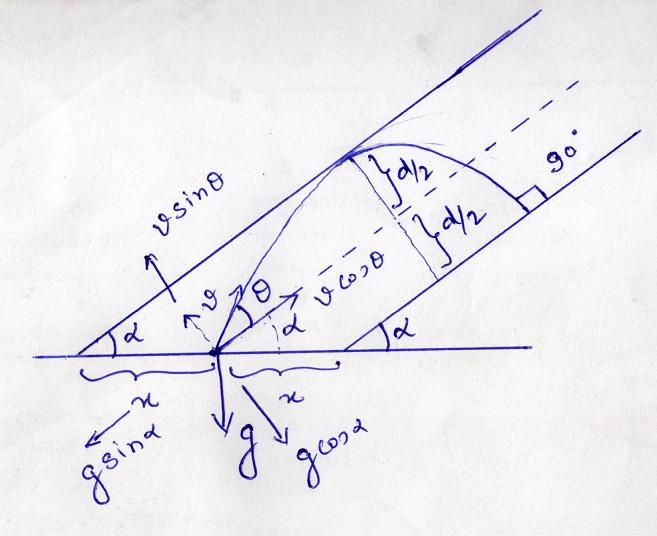# Projectile

2 parallel st. lines are inclined to the horizontal at an angle Î± .A particle is projected from a point midway between them so as to graze one of the linesand strike the other at right angle .If Î¸ be the nagle btwn direction of projection and either of the lines,then tanÎ¸ = (√2 -1)cotÎ± .

Plz provide solution as well

•Akash Anand ·

For now just take a hint. See the diagram and try to understand the equation that I m providing. You have to think the reason for every equation, and finally solve them to get the result.
These are the following equation you need for getting your result.

(u sinÎ¸)22g cosÎ±=d2.....(i)

T=u cosÎ±g sinÎ±........(ii)
-d2=u sinÎ¸*T-12*g cosÎ±T2.........(iii)

Try to put the values from eq. (i) & (ii) in (iii) and solve.Akshay Ginodia ·

angle*

•Akshay Ginodia ·

Thank u sir :)

•